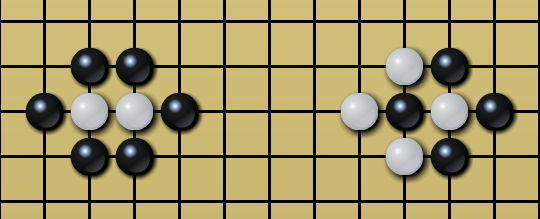시간 제한 메모리 제한 제출 정답 맞은 사람 정답 비율
6 초 512 MB 0 0 0 0.000%

## 문제

Go is an adversarial game with the objective of surrounding a larger total area of the board with one's stones than the opponent's. The core idea of the game is the concept of liberty, which is an open point, or rather, an intersection of vertical and horizontal lines on the chessboard with no stones on it, bordering the group.

A stone, white or black, is called alive if it has at least one liberty directly orthogonally adjacent (up, down, left, or right), or must be in the same connected group with a stone of the same color which is alive. We say two stones of the same color are directly connected if they're orthogonally adjacent. We say two stones $s_1$ and $s_k$ of the same color are in the same connected group if there exists a sequence of stones $s_1, s_2,\cdots, s_k$ such that for all $1 \le i < k$, $s_{i-1}$ and $s_i$ are of the same color and are directly connected.

For example, in the left part of the below figure, neither of the two white stones is alive, as they're captured by the surrounding black stones; While in the right part, the rightmost white stone is also not alive, even if the leftmost black stone is also not alive.Given a chessboard with $n$ vertical and $n$ horizontal lines where some stones might be lying on, please calculate the number of white stones captured by the black ones (that is to say, calcaulate the number of white stones not alive). The results for the above examples are $2$ and $1$, respectively.

However, our dear friend Kotori thinks that this problem is too simple for our clever contestants to solve, so she would like to heat things up by instead asking you to flip the color of each stone (that is to say, change a black stone to a white one, or vice versa1) independently and find out the corresponding answer after each flip.

By flipping independently we mean that before flipping the color of a stone, the other stones should change back to their original color. Also note that the data in this problem is not from the real world, which means that the size of the chessboard is not necesssarily $19 \times 19$, and the number of white and black stones can be any integer.

1Vice versa: The reverse is also true. Here it can be replaced with "change a white stone to a black one". This is a very common phrase in modern English especially in academic writing, so please bear it in mind.

## 입력

There are multiple test cases. The first line of the input contains an integer $T$ indicating the number of test cases. For each test case:

The first line contains one integer $n$ ($2\le n \le 10^3$) indicating the length of the board side.

For the next $n$ lines, the $i$-th line contains a string $s_{i,1},s_{i,2},\cdots,s_{i,n}$ ($s_{i,j}$ $\in$ $\{\text{'x' (ascii: 120)}$, $\text{'o' (ascii: 111)}$, $\text{'.' (ascii: 46)}\}$), where $s_{i,j} = \text{'x'}$ indicates that the intersection on the $i$-th row and the $j$-th column contains a black stone. Similarly $s_{i, j} = \text{'o'}$ for a white stone and $s_{i,j} = \text{'.'}$ for an empty intersection.

It's guaranteed that the sum of $n$ over all test cases does not exceed $5 \times 10^3$.

## 출력

For each test case output an integer $E$ modulo $(10^9 + 7)$ which is the answer encoded as follows:

• Sort all the stones with their row number (from top to bottom) as the primary sort key and their column number (from left to right) as the secondary sort key;
• $E=\sum \limits_{i=1}^m (10^6 + 7)^{m-i}a_i$, where $m$ is the number of stones and $a_i$ is the number of white stones not alive after flipping the color of the $i$-th stone.

NOTE that the MODULUS and the BASE are DIFFERENT. (We're begging you to notice this sentence. If this is not a pdf file I would rather it flashes and twinkles like crazy.

## 예제 입력 1

3
2
.o
..
3
.x.
xoo
ox.
2
oo
oo


## 예제 출력 1

0
870527216
485539347


## 힌트

For the second sample test case, after flipping the stones in the order of $(1,2)$, $(2,1)$, $(2,2)$, $(2,3)$, $(3,1)$, $(3,2)$, the number of dead white stones are $1$, $0$, $1$, $2$, $0$, $0$, repectively.

For the third sample test case all stones on the chessboard, black or white, are not alive.